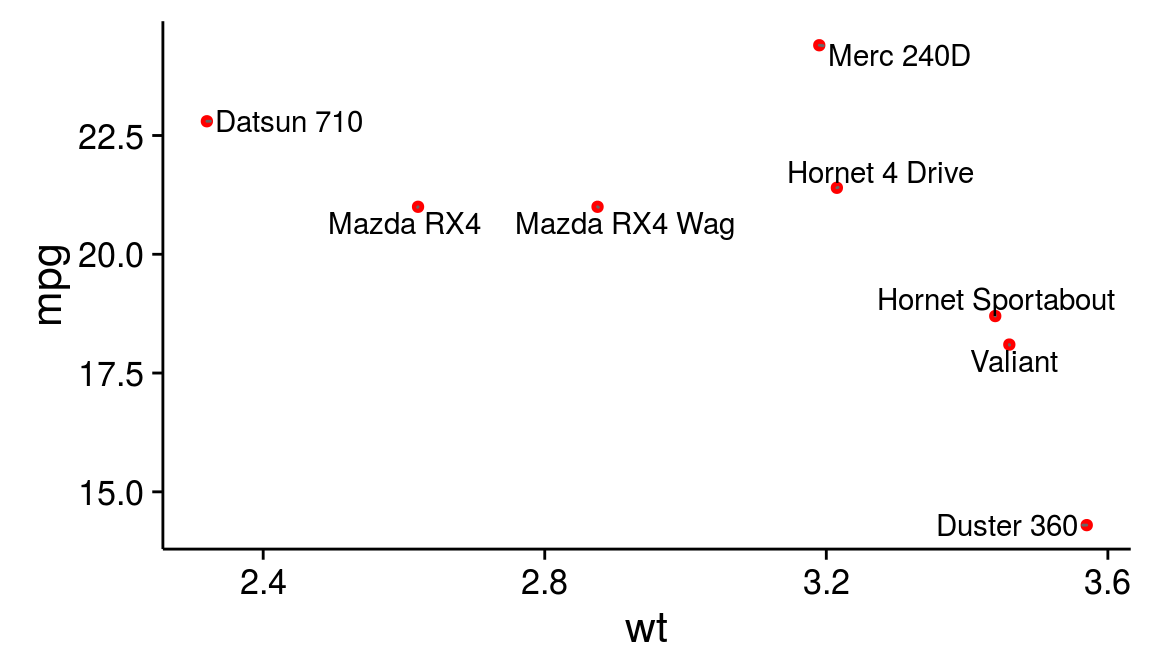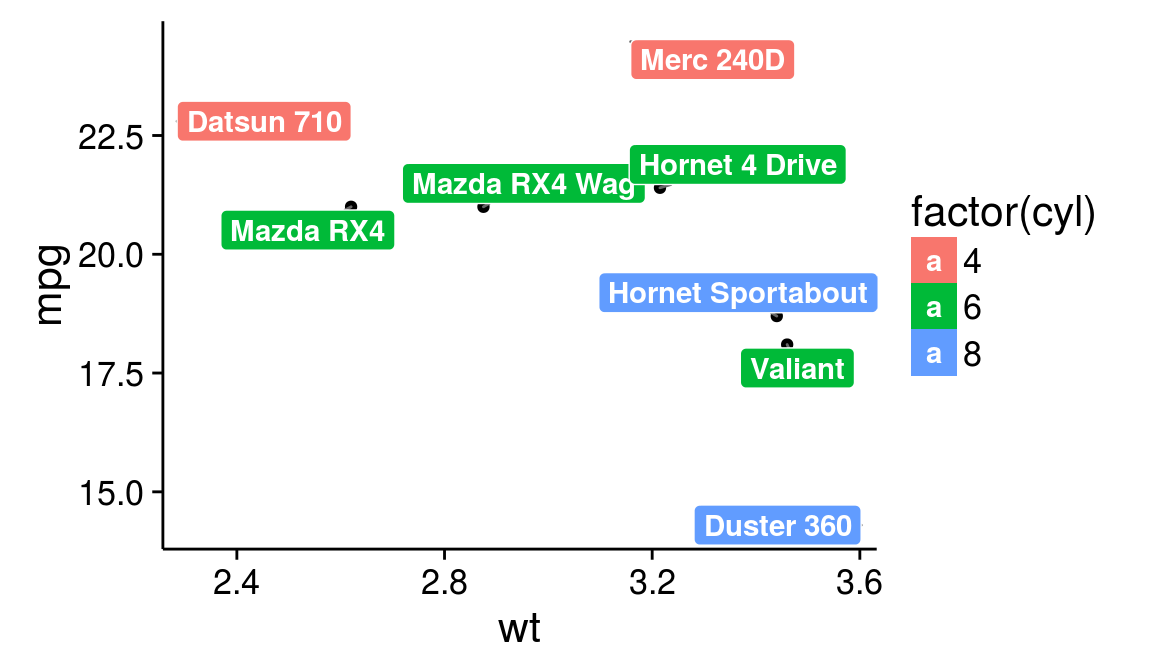ggplot2 now has an official extension mechanism. This means that others can now easily create their own stats, geoms and positions, and provide them in other packages. This should allow the ggplot2 community to flourish, even as less development work happens in ggplot2 itself. This page showcases these extensions.

### ggrepel

https://github.com/slowkow/ggrepel

Repel overlapping text labels away from each other.

``````# Example from https://github.com/slowkow/ggrepel
library(ggplot2)
library(ggrepel)

## We can repel the text labels away from each other by loading ggrepel and using geom_text_repel instead:
set.seed(42)
dat <- mtcars[1:8,]
ggplot(dat) +
geom_point(aes(wt, mpg), color = 'red') +
geom_text_repel(aes(wt, mpg, label = rownames(dat))) +
theme_classic(base_size = 16)````````````#geom_label_repel is based on geom_label.
set.seed(42)
ggplot(dat) +
geom_point(aes(wt, mpg)) +
geom_label_repel(
aes(wt, mpg, fill = factor(cyl), label = rownames(dat)),
fontface = 'bold', color = 'white',